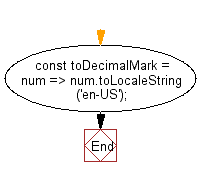# JavaScript: Convert a float-point arithmetic to the Decimal mark form and It will make a comma separated string from a number

## JavaScript: Exercise-125 with Solution

Write a JavaScript program to convert a float-point arithmetic to the Decimal mark form and It will make a comma separated string from a number.

• Use Number.prototype.toLocaleString() to convert the number to decimal mark format.

Sample Solution:

JavaScript Code:

``````//#Source https://bit.ly/2neWfJ2
const toDecimalMark = num => num.toLocaleString('en-US');
console.log(toDecimalMark(12305030388.9087));
console.log(toDecimalMark(123.2264))
console.log(toDecimalMark(-100.10))
```
```

Sample Output:

```12,305,030,388.909
123.226
-100.1
```

Flowchart:Live Demo:

See the Pen javascript-basic-exercise-125-1 by w3resource (@w3resource) on CodePen.

Improve this sample solution and post your code through Disqus

What is the difficulty level of this exercise?

Test your Programming skills with w3resource's quiz.

﻿

## JavaScript: Tips of the Day

Chunks an array into n smaller arrays

Example:

```const tips_chunkIntoN = (arr, n) => {
const size = Math.ceil(arr.length / n);
return Array.from({ length: n }, (v, i) =>
arr.slice(i * size, i * size + size)
);
}
console.log(tips_chunkIntoN([1, 2, 3, 4, 5, 6, 7,8], 4));
```

Output:

```[[1,2],[3,4],[5,6],[7,8]]
```